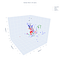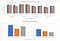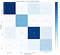# Introduction

Isotope signatures of methane is an important indicator for gas provenance. Previously in this field, people have already classified natural methane gases into thermogenic, abiotic, and biogenic categories by their bulk δ13C and δD values, but there are two problems for this classification method: first, the boundaries are drawn empirically, so they may not generate the best separation results; second, there is significant overlap between three categories in this two-dimension space, so some data points may be misinterpreted. Recently, clumped isotope signature Δ13CH3D and Δ12CH2D2 have proven to be a good indicator for the source of methane as well. If we also include clumped isotope signatures as features in our model, the classification result can be improved. Here we present a multi-class classification of methane gases based on their isotope signatures. Since there are few data for Δ12CH2D2 so far, and some samples may have mixed sources, we use δ13C, δD and Δ13CH3D as the three features in our model, and thermogenic, abiotic, biogenic, mixed thermogenic and microbial (mixed T-M) as our four target classes. We make a comparison between two machine learning algorithms: support vector classification and neural network, and then compare between using only two features (δ13C and δD) and three features (δ13C, δD, and Δ13CH3D). The data we use in this study comes from a compilation of 350 samples from recent research papers.

# Method

Data visualization

We visualize the data points in δ13C-δD and Δ13CH3D-δ13C-δD space (Fig.1). There is significant overlapping between four target classes in 2D space (Fig. 1, left panel). The abiotic and mixed T-M data points are hardly distinguishable from biogenic and thermogenic ones. In 3D space, four classes are more distinguishable (Fig. 1, right panel), but abiotic data points are still quite scattered and overlap with other classes. Mixed T-M strongly overlaps with thermogenic and biogenic classes as well, which is reasonable as these samples are mixtures of two endmembers. We first standardize the data, then randomly split them into training (85%) and testing sets (15%).Figure 1. Standardized data points in 2D (top) and 3D spaces (bottom). Feature 1, feature 2, and feature 3 are δD, δ13C, and Δ13CH3D, respectively.

Support vector classification

We use the SVM package from sklearn to do support vector classification (SVC) for our data. Within SVC, we tried radial basis function (RBF), linear, polynomial, and sigmoid kernels, and the results show that for the same C and gamma values, RBF kernel gives the best fit for both training set and testing set, so we will only show the results from RBF kernel in later discussions. To get the best fit, we use loops to search for C and gamma values that give the highest accuracy for training and test set on average:

`acc_test = []for l in np.arange(1,101):svc_rbf = svm.SVC(kernel=’rbf’, C = l)  svc_rbf.fit(X_train, np.ravel(y_train))  y_pred_svc_rbf = svc_rbf.predict(X_test)  avg_acc = accuracy(y_pred_svc_rbf, np.ravel(y_test))   + accuracy(svc_rbf.predict(X_train), np.ravel(y_train))  acc_test.append(avg_acc)print(‘Index for the first occurence of maximum:’, np.argmax(acc_test))print(acc_test[np.argmax(acc_test)])C_max = 1 + np.argmax(acc_test)`

The optimal C and gamma values differ between each run, but C is generally around 10, and gamma is around 3, so we choose C=10 and gamma=3 as the model parameter.

In addition to the three-feature model, we also train our support vector classifier with only two features: δ13C and δD, to evaluate whether the inclusion of Δ13CH3D will improve the classification.

Classification by neural network

To make a comparison between different machine learning algorithms, we train a fully connected neural network with PyTorch. Because our training set is very small, the number of samples can not support a neural network with a hidden layer size that is too large. After some experiments, we find a model with 2 hidden layers and 6 neurons in each layer that give the best fit for our data. With our 3 input features and 4 output classes, the total number of model parameters is 94. The activation functions are another factor we have to consider. As our goal is to classify the data, the activation function of the output layer should be a softmax function. For the activation function of two hidden layers, between sigmoid and ReLU function, sigmoid gives a slightly better result during several runs, so we choose it as the activation function for hidden layers:

`model = torch.nn.Sequential( torch.nn.Linear(D_in, H1), torch.nn.Sigmoid(), torch.nn.Linear(H1, H2), torch.nn.Sigmoid(), torch.nn.Linear(H2, D_out), torch.nn.Softmax(dim = 1))`

For softmax regression classifier, a cross-entropy loss is usually used:

`loss_fn = torch.nn.CrossEntropyLoss(reduction = ‘mean’)`

We apply adam optimizer with a learning rate of 1e-3. This learning rate has proved to be the optimal choice between training speed and accuracy. The learning rate of 1e-2 is too large to converge to the global optimum; while 1e-4 is too small so that the training time is too long (usually takes more than 100,000 iterations), and sometimes the model is stuck at a sub-optimal solution due to the small steps in each iteration.

Another approach we take to balance the training speed and accuracy is setting a threshold value for loss reduction every 10,000 iterations, instead of stopping the loop at a fixed iteration number. Since the total number of our samples is small, the randomness in train-test splitting has a relatively large influence on the iteration numbers it takes to converge. We choose 1e-4 as our threshold for loss reduction, as too small the threshold would result in overfitting and slow training speed, and too large the threshold would reduce the accuracy of the model. It usually takes 30,000 to 50,000 iterations for the model to converge given the threshold of 1e-4. To speed up the training, we run the code on GPU:

`device = torch.device("cuda:0")while deltaL >= 1e-4 or deltaL < 0:   y_pred = model(x)   loss = loss_fn(y_pred, y)   if t % 10000 == 9999:     print(‘iteration number:’, t)     print(‘cross entropy loss:’, loss.item())     deltaL = loss_prev — loss.item()     loss_prev = loss.item()   t += 1   optimizer.zero_grad()   loss.backward()   optimizer.step()`

These approaches enable us to train the model within one minute while keeping the accuracy on the testing set above 80 %.

# Model evaluation and discussion

First, we compare the precision, recall, and accuracy of three models: neural network, support vector classifier with three features, and two features (Fig. 2). The result may be different for each run because of the random splitting, but it follows a general pattern. Here we show the result of one individual run.Figure 2. Comparison of precision, recall, and accuracy between the three models.

Between support vector classifier and neural network, the former one gives a better training result for our dataset, with the same or better performance in all error metrics we use in this study. In this run, the accuracy of the support vector classifier on the training set is 97.0 % and on the testing set is 88.9 %. Recall and precision are all above 0.8, except for the precision of mixed T-M class, which is 0.71. Furthermore, it only takes a few seconds to train an SVC, compared with a nearly one-minute training time for a neural network. This shows the capability of support vector machine in fitting small datasets, with shorter training time, better fit, and proof against overfitting. While neural network is a powerful algorithm, it is not the best choice in this study.

Then we further evaluate the performance of SVC with three features and two features. In this case, the difference in training time is negligible. Results show that the support vector classifier with three features is better than with two features, giving a higher precision and recall for thermogenic class, and a significantly higher accuracy on the training set and testing set. This indicates that the involvement of Δ13CH3D as an extra feature can improve the overall performance of the model.Figure 3. Confusion Matrix of Support Vector Classifier with Three Features

To go into details for the distribution of errors, we plot the confusion matrix for the support vector classifier with three features (Fig. 3). Surprisingly, the abiotic class, which looks quite scattered in 3D space, has the best classification result in this run. Some biogenic and thermogenic data points are mistaken for other classes, which is quite reasonable since these two classes have more data points and a few of them are erratic. To demonstrate the solution, we also plot the support vectors in our model (Fig. 4).